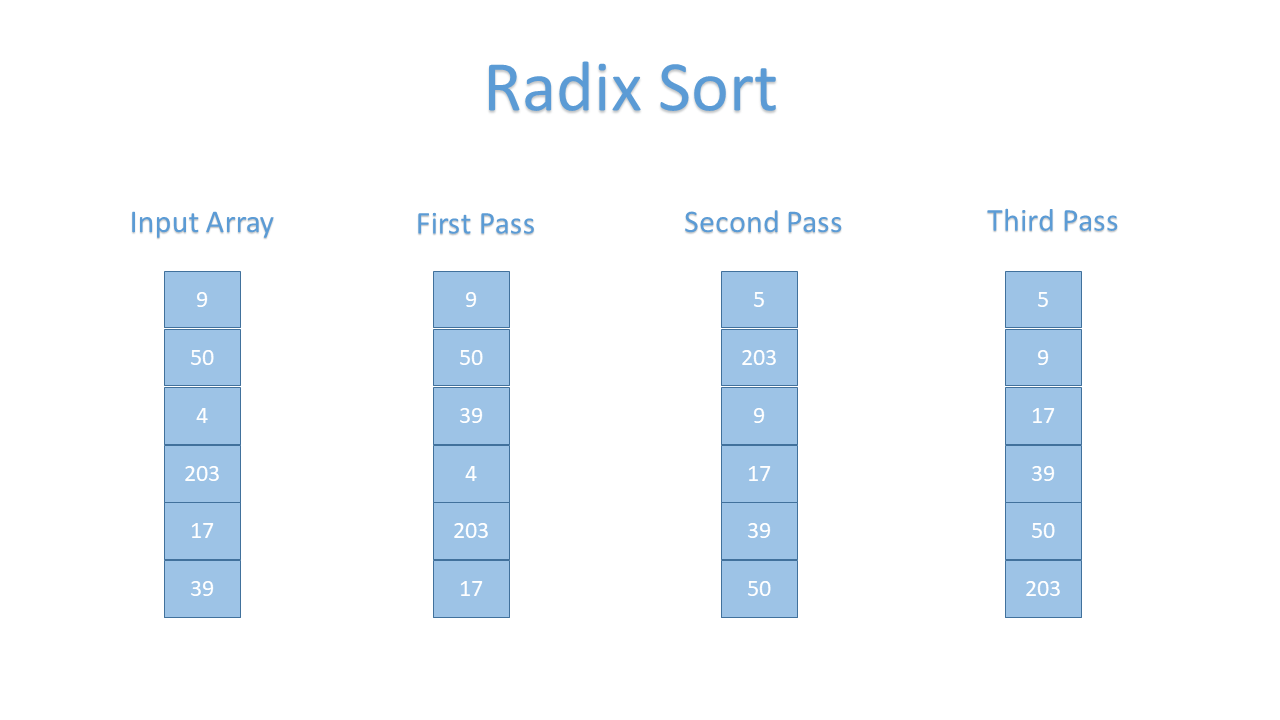Sheeraz Gul Apr 04, 2022

In `Radix Sort`, the elements are sorted by first grouping the individual numbers of the same place value and then sorted according to the ascending or descending order. This tutorial explains the `radix sort` algorithm in detail and demonstrates the implementation of radix sort in Java.

Follow the steps below to apply the `radix sort`.

1. First of all, find the maximum element from the input array; that maximum number will then be used to go through the significant places of all array members.
2. Next, go through each significant place one by one. We can use any stable sorting algorithm, for example, the counting sort, to sort the elements of each significant place.

Support an array of six elements. The `radix sort` will first sort the elements based on the values of the unit place.

Then sorts the elements of the array based on the value of the tenth place.

Suppose the array is `[9, 50, 4, 203, 17, 39]`. The picture below shows this array sorted according to the `radix sort` with multiple passes.### Time Complexity of Radix Sort Algorithm

The table below shows the time complexity of the `radix sort` algorithm in different cases.

Time Complexity Case
`Ω(n+k)` Best Case
`θ(nk)` Average Case
`O(nk)` Worst Case
1. Best Case - When no sorting is required, the array is already sorted. In the Best case scenario, the `radix sort` time complexity is `Ω(n+k)`.
2. Average Case - The array elements are in a chaotic order, not properly descending or ascending order. The `Radix Sort` time complexity is `θ(nk)` in the average case scenario.
3. Worst-Case - When the array elements must be sorted in reverse order, for example, from ascending to descending or descending to ascending order. The `Radix Sort` time complexity is `O(nk)` in the worst-case scenario.

### Pseudo Code of Radix Sort Algorithm

The Pseudo Code for the `Radix Sort` algorithm is given below.

``````Radix_Sort(Input_Array)
MAX = largest number in the input array
DIGIT = number of digits in the largest number
Now, create DIGIT buckets of size 0 - 9
for x -> 0 to DIGIT
sort the elements according to any stable sort
``````

## Radix Sort Algorithm Implementation in Java

Using the `counting sort`, let’s implement the `radix sort` algorithm. See example:

``````package delftstack;

import java.util.Arrays;

public static void main(String args[]) {
int[] input_array = { 9, 50, 4, 203, 17, 39};
int array_size = input_array.length;
System.out.println("Sorted Array Using Radix Sort: ");
System.out.println(Arrays.toString(input_array));
}

// Counting sort to sort the elements on the basis of significant places
void Counting_Sort(int input_array[], int array_size, int number_place) {
int[] output_array = new int[array_size + 1];
int max_number = input_array;
for (int x = 1; x < array_size; x++) {
if (input_array[x] > max_number) {
max_number = input_array[x];
}
}
int[] count_array = new int[max_number + 1];

for (int x = 0; x < max_number; ++x) {
count_array[x] = 0;
}
// Calculating the count of elements
for (int x = 0; x < array_size; x++) {
count_array[(input_array[x] / number_place) % 10]++;
}
// Calculating the cumulative count
for (int x = 1; x < 10; x++) {
count_array[x] += count_array[x - 1];
}
// Sorting the elements
for (int x = array_size - 1; x >= 0; x--) {
output_array[count_array[(input_array[x] / number_place) % 10] - 1] = input_array[x];
count_array[(input_array[x] / number_place) % 10]--;
}

for (int x = 0; x < array_size; x++) {
input_array[x] = output_array[x];
}
}

// Get the largest element from input array
int Get_Max(int input_array[], int array_size) {
int max_number = input_array;
for (int i = 1; i < array_size; i++) {
if (input_array[i] > max_number) {
max_number = input_array[i];
}
}
return max_number;
}

void Radix_Sort(int input_array[], int array_size) {

// Get the maximum number
int max_number = Get_Max(input_array, array_size);

// Apply the counting sort based on significant place value.
for (int number_place = 1; max_number / number_place > 0; number_place *= 10) {
Counting_Sort(input_array, array_size, number_place);
}
}
}
``````

The code above implements the radix sort with the help of the `counting sort`. See output:

``````Sorted Array Using Radix Sort:
[4, 9, 17, 39, 50, 203]
``````
Author: Sheeraz Gul

Sheeraz is a Doctorate fellow in Computer Science at Northwestern Polytechnical University, Xian, China. He has 7 years of Software Development experience in AI, Web, Database, and Desktop technologies. He writes tutorials in Java, PHP, Python, GoLang, R, etc., to help beginners learn the field of Computer Science.